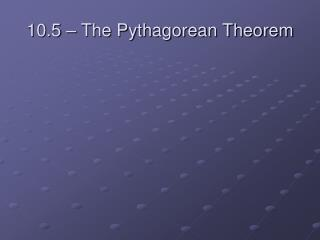DownloadDownload Presentation10.5 – The Pythagorean Theorem

# 10.5 – The Pythagorean Theorem

Télécharger la présentation## 10.5 – The Pythagorean Theorem

- - - - - - - - - - - - - - - - - - - - - - - - - - - E N D - - - - - - - - - - - - - - - - - - - - - - - - - - -
##### Presentation Transcript

1. 10.5 – The Pythagorean Theorem

2. leg leg

3. hypotenuse leg leg

4. hypotenuse leg leg Pythagorean Theorem: For a right triangle with legs a and b and hypotenuse c, then a2 + b2 = c2, where a is the smaller of the two legs.

5. Ex. 1 Find the length of the missing side. a 25 10

6. Ex. 1 Find the length of the missing side. a 25 10 a2 + b2 = c2

7. Ex. 1 Find the length of the missing side. a 25 10 a2 + b2 = c2 a2 + (10)2 = (25)2

8. Ex. 1 Find the length of the missing side. a 25 10 a2 + b2 = c2 a2 + (10)2 = (25)2 a2 + 100 = 625

9. Ex. 1 Find the length of the missing side. a 25 10 a2 + b2 = c2 a2 + (10)2 = (25)2 a2 + 100 = 625 a2 = 525

10. Ex. 1 Find the length of the missing side. a 25 10 a2 + b2 = c2 a2 + (10)2 = (25)2 a2 + 100 = 625 a2 = 525 a ≈ 22.91

11. Ex. 2 Determine whether the following side measures form right triangles. a. 20, 21, 29

12. Ex. 2 Determine whether the following side measures form right triangles. a. 20, 21, 29 Shorter lengths must be the legs.

13. Ex. 2 Determine whether the following side measures form right triangles. a. 20, 21, 29 Shorter lengths must be the legs. So, a2 + b2 = c2

14. Ex. 2 Determine whether the following side measures form right triangles. a. 20, 21, 29 Shorter lengths must be the legs. So, a2 + b2 = c2 (20)2 + (21)2 = (29)2

15. Ex. 2 Determine whether the following side measures form right triangles. a. 20, 21, 29 Shorter lengths must be the legs. So, a2 + b2 = c2 (20)2 + (21)2 = (29)2 400 + 441 = 841

16. Ex. 2 Determine whether the following side measures form right triangles. a. 20, 21, 29 Shorter lengths must be the legs. So, a2 + b2 = c2 (20)2 + (21)2 = (29)2 400 + 441 = 841 841 = 841

17. Ex. 2 Determine whether the following side measures form right triangles. a. 20, 21, 29 Shorter lengths must be the legs. So, a2 + b2 = c2 (20)2 + (21)2 = (29)2 400 + 441 = 841 841 = 841 , so they form a rt. triangle!